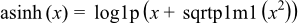Boost C++ Libraries

...one of the most highly regarded and expertly designed C++ library projects in the world.

This is the documentation for an old version of boost. Click here for the latest Boost documentation.

asinh

#include <boost/math/special_functions/asinh.hpp>

template<class T>
calculated-result-type asinh(const T x);

template<class T, class Policy>
calculated-result-type asinh(const T x, const Policy&);

Computes the reciprocal of the hyperbolic sine function.

The return type of this function is computed using the result type calculation rules: the return type is double when T is an integer type, and T otherwise.The final Policy argument is optional and can be used to control the behaviour of the function: how it handles errors, what level of precision to use etc. Refer to the policy documentation for more details.

Accuracy

Generally accuracy is to within 1 or 2 epsilon across all supported platforms.

Testing

This function is tested using a combination of random test values designed to give full function coverage computed at high precision using the "naive" formula:along with a selection of sanity check values computed using functions.wolfram.com to at least 50 decimal digits.

Implementation

For sufficiently large x we can use the approximation:While for very small x we can use the approximation:For 0.5 > x > ε the following rearrangement of the primary definition is used:Otherwise evalution is via the primary definition: